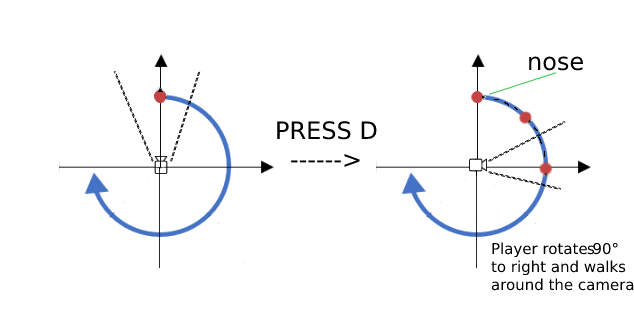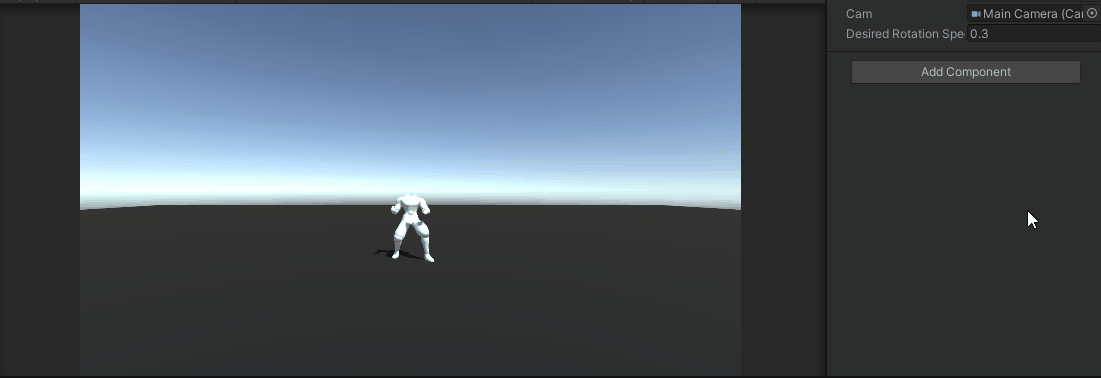# Rotation movement

i used this code to rotate the player based on where it goes

``````if (movementDirection != Vector3.zero)
{
Quaternion toRotation = Quaternion.LookRotation(movementDirection, Vector3.up);
transform.rotation = Quaternion.RotateTowards(transform.rotation, toRotation, rotationSpeed * Time.deltaTime);
}
``````

Now i need to rotate the player for a certain angle if it moves on the x (input: horizontal).

``````if (movementDirection.x != 0) { /*abracadabra*/ }
``````

_
So I have the camera in the middle and the player that moves around as it moves:(red is your camera, green is player)

``````Transform subject = player.transform;
Vector3 initialPosRelative = subject.position - pivot;
Quaternion initialRotation = subject.rotation;
Vector3 pivot = cameraTransform.position;
Vector3 axis  =  cameraTransform.up;// < < axis of rotation
float rotationSpeed = 10f;
float t = rotationSpeed * Time.deltaTime;
Quaternion rot = Quaternion.AngleAxis( angle * t , axis );

subject.position = pivot + rot * initialPosRelative;
subject.rotation = initialRotation * rot;
``````

Code is not tested but you should get the idea: rotate relative position around an axis.
_

I don’t think we understood each other …
or I’m the one who doesn’t understand what you showed me
but I need my third person character to be able as he goes left or right to walk a path in a circle

As you see in the image:
in the middle there is the camera and my character who goes to the right walks facing right “around the room that looks at him” @andrew-lukasik@MicheleSurgo
Well, give this one a try. This time, you’ll have to create an empty child gameobject of the camera, and remember to handle that animator part.

``````Vector3 desiredMoveDirection;
Vector3 lastMoveDirection;
Transform camLock;

public Camera cam;
public Transform camChild;

public float desiredRotationSpeed;

float distance;
bool isTurning;

void Update()
{
//Make the camChild y position the same as the player
if (!isTurning)
camChild.transform.position = new Vector3(cam.transform.position.x, transform.position.y, cam.transform.position.z);
float horizontalInput = Input.GetAxisRaw("Horizontal");
float verticalInput = Input.GetAxisRaw("Vertical");

Vector3 movementDirection = new Vector3(horizontalInput, 0, verticalInput);

var forward = cam.transform.forward;
var right = cam.transform.right;

forward.y = 0f;
right.y = 0f;

forward.Normalize();
right.Normalize();

if (movementDirection != Vector3.zero)
{
desiredMoveDirection = forward * movementDirection.z + right * movementDirection.x;
lastMoveDirection = desiredMoveDirection;
//When the player does press W or S
if (verticalInput != 0)
{
Quaternion toRotation = Quaternion.LookRotation(lastMoveDirection, Vector3.up);
transform.rotation = Quaternion.Slerp(transform.rotation, toRotation, desiredRotationSpeed);

}

//Test when the player press D
else
{
if (Input.GetKey(KeyCode.D) && !isTurning)
{
//Turn
camLock = camChild.transform;
distance = (transform.position - camLock.position).magnitude;
newRotation = new Vector3(camChild.transform.eulerAngles.x, camChild.transform.eulerAngles.y + 90, camChild.transform.eulerAngles.z);
isTurning = true;
StartCoroutine(TurnRight());
}
}
}

anim_Warrior(movementDirection);
}

Vector3 newRotation;

IEnumerator TurnRight()
{
while (camChild.transform.rotation != Quaternion.Euler(newRotation))
{
//Rotate the camChild gameObject to set the path for the player
camChild.transform.SetPositionAndRotation(camLock.position, Quaternion.Lerp(camChild.transform.rotation, Quaternion.Euler(newRotation), Time.deltaTime));
//make the player rotate and move in a curve path
transform.SetPositionAndRotation(camChild.transform.position + camChild.transform.forward * distance, Quaternion.LookRotation(camChild.transform.right));

//Write some code here to make the animator play that walking animation
//Such as anim_Warrior(movementDirection) but now the movementDirection is now zero so try something else
yield return null;
}
isTurning = false;
transform.rotation = Quaternion.LookRotation(transform.right * -1);

//Do something to stop walking the animation
}
``````

No that’s not what I’m saying … @eneIr

This is my movement code. The player have 3 functions (Rotate to direction, Rotate to camera facing, “Curve if it goes horizontal”

But now i have another problem … First play it works… after my player doesnt move. IDK

``````void Update()
{

float horizontalInput = Input.GetAxisRaw("Horizontal");
float verticalInput = Input.GetAxisRaw("Vertical");

Vector3 movementDirection = new Vector3(horizontalInput, 0, verticalInput);

var camera = Camera.main;
var forward = cam.transform.forward;
var right = cam.transform.right;

forward.y = 0f;
right.y = 0f;

forward.Normalize();
right.Normalize();

desiredMoveDirection = forward * movementDirection.z + right * movementDirection.x;

if (movementDirection != Vector3.zero)
{

lastMoveDirection = desiredMoveDirection;

}

Quaternion toRotation = Quaternion.LookRotation(lastMoveDirection, Vector3.up);
transform.rotation = Quaternion.Slerp(transform.rotation, toRotation, desiredRotationSpeed);

// THIS IS THE CODE I AM TRYING TO DO
// IF horizontalInput the player walks turning
// so it doesn't go straight but it takes a curve

/*if(movementDirection.x != 0)
{
Vector3 angleRotation = new Vector3(0, angle, 0);
transform.transform.Rotate(angleRotation, Space.World);
}*/

anim_Warrior(movementDirection);
}
``````

This is what happens @eneIr
sorry for my headless player. I didn’t want to scandalize anyone …@MicheleSurgo Well, give this one a try. This time, you’ll have to create an empty child gameobject of the camera, and remember to handle that animator part.

``````         Vector3 desiredMoveDirection;
Vector3 lastMoveDirection;
Transform camLock;

public Camera cam;
public Transform camChild;

public float desiredRotationSpeed;

float distance;
bool isTurning;

void Update()
{
//Make the camChild y position the same as the player
if (!isTurning)
camChild.transform.position = new Vector3(cam.transform.position.x, transform.position.y, cam.transform.position.z);
float horizontalInput = Input.GetAxisRaw("Horizontal");
float verticalInput = Input.GetAxisRaw("Vertical");

Vector3 movementDirection = new Vector3(horizontalInput, 0, verticalInput);

var forward = cam.transform.forward;
var right = cam.transform.right;

forward.y = 0f;
right.y = 0f;

forward.Normalize();
right.Normalize();

if (movementDirection != Vector3.zero)
{
desiredMoveDirection = forward * movementDirection.z + right * movementDirection.x;
lastMoveDirection = desiredMoveDirection;
//When the player does press W or S
if (verticalInput != 0)
{
Quaternion toRotation = Quaternion.LookRotation(lastMoveDirection, Vector3.up);
transform.rotation = Quaternion.Slerp(transform.rotation, toRotation, desiredRotationSpeed);

}

//Test when the player press D
else
{
if (Input.GetKey(KeyCode.D) && !isTurning)
{
//Turn
camLock = camChild.transform;
distance = (transform.position - camLock.position).magnitude;
newRotation = new Vector3(camChild.transform.eulerAngles.x, camChild.transform.eulerAngles.y + 90, camChild.transform.eulerAngles.z);
isTurning = true;
StartCoroutine(TurnRight());
}
}
}

anim_Warrior(movementDirection);
}

Vector3 newRotation;

IEnumerator TurnRight()
{
while (camChild.transform.rotation != Quaternion.Euler(newRotation))
{
//Rotate the camChild gameObject to set the path for the player
camChild.transform.SetPositionAndRotation(camLock.position, Quaternion.Lerp(camChild.transform.rotation, Quaternion.Euler(newRotation), Time.deltaTime));
//make the player rotate and move in a curve path
transform.SetPositionAndRotation(camChild.transform.position + camChild.transform.forward * distance, Quaternion.LookRotation(camChild.transform.right));

//Write some code here to make the animator play that walking animation
//Such as anim_Warrior(movementDirection) but now the movementDirection is now zero so try something else
yield return null;
}
isTurning = false;
transform.rotation = Quaternion.LookRotation(transform.right * -1);

//Do something to stop walking the animation
}
``````